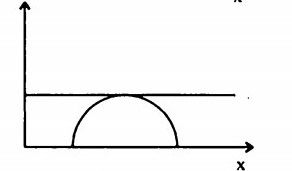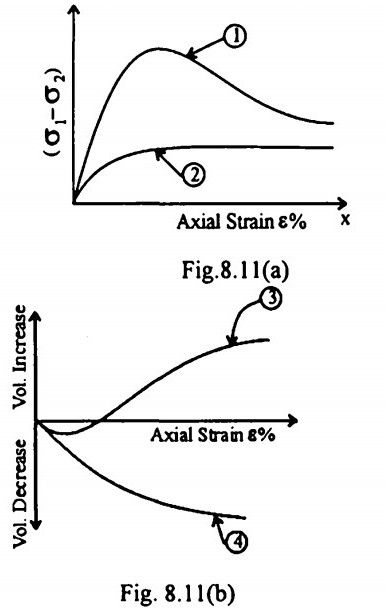top of pageSearch

# Soil Mechanics (241-260)

Updated: Apr 27, 2020

241. A sample of clay and a sample of sand have the same specific gravity and void

ratio. Their permeabilities would differ because the size ranges of their voids would be different

242. During seepage through an earth mass, the direction of seepage is

perpendicular to the equipotential lines

243. Consider the following limitations :

1. Plane of failure is predetermined

2. There is virtually no control on drainage /

3. Non-uniform distribution of stresses The limitation inherent in direct shear test include :

244. Consider the following assumptions:

1. Failure occurs on a plane surface.

2. Failure wedge is a rigid body

Coulomb's theory of earth pressure is based on assumptions

245. In a saturated clay layer undergoing consolidation with single drainage at its

top, the pore water pressure would be the maximum at its bottom

246. A saturated clay stratum of thickness 10 m, bounded on top and bottom by medium coarse sand layers, has a coefficient of consolidation of 0.002 cm²/s. If this stratum is subjected to loading, it is likely that it would undergo 50% of its primary consolidation in 284 days

247. The stress distribution at a depth beneath a loaded area is determined using

Newmark's influence chart which indicate an influence value of 0.005. The number of the segments covered by the loaded area in the chart is 20 and the intensity of loading on the area is 10 T/m². The intensity of stress distribution at that depth is 1 T/m²

248. Consider the following field tests :

2. Instrumented test pile

While estimating the load carrying capacity of a pile, the tests that can be

used for separating the skin resistance from point resistance, would include

249. The difference between maximum void ratio and minimum void ratio of a sand sample is 0.30. If the relative density of this sample is 66.6% at a void ratio of 0.40, then the void ratio of this sample at its loosest state will be 0.60

250. A particular soil sample is subjected to test for the determination of permeability coefficient in two separate constant head permeameters, whose specifications are as under:If the tests on both the permeameters are conducted with equal head of water applied on the samples, then the ratio of amount of water discharged through the permeameters A & B during a period of one hour will be 0.125

251. The foundation soil under the toe of a dam has a void ratio V . The specific gravity of the soil solid is G. Factor of safety against piping is to be taken as 2.5. The maximum permissible upward exit gradient is given by252. A homogeneous earth dam with no horizontal drainage filter at downstream is shown in Fig.8.8. The slope of the downstream side, β is less than 30°. In

order to determine the value of 'a1, the discharge 'q' per unit length through the

section of height a sin β is assumed to be (k= coefficient of permeability of soil)ka sin β tan β

253. Consider the flow net shown in Fig.8.9 :The ratio of the number of flow channels to the number of potential drops is

3/7

254. Which one of the following parameters can be used to estimate the angle of

internal friction of a sandy soil? density index

255. Which one of the following diagrams (Fig) correctly illustrates the Mohr's

stress conditions of unconfined shear test on cohesive soil ? ( x-axis Normal

stress; y-axis Shear stress).256. A CD triaxial test is performed on a clay soil. Figures 8.11(a) and (b) show two

curves each for deviator stress v/s axial strain % and volume change v/s axial

strain %. If the clay is overconsolidated, then the results would be as in curves1 and 3

257. A cantilever sheet pile derives its stability from lateral resistance of soil

258.Deflection of a sheet pile in a braced cut increases from top to bottom

259. A completely saturated normally consoli dated clay is tested in a triaxial test under consolidated undrained condition. The value of pore pressure coefficient at

failure, Af is given by (Δσ₃=change in cell pressure; Δσ₁,= change in axial stress; Δu=

corresponding change in pore pressure)260. The time V required for attaining a certain degree of consolidation of a clay

layer is proportional to H² and l/C